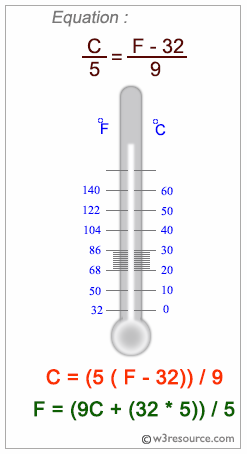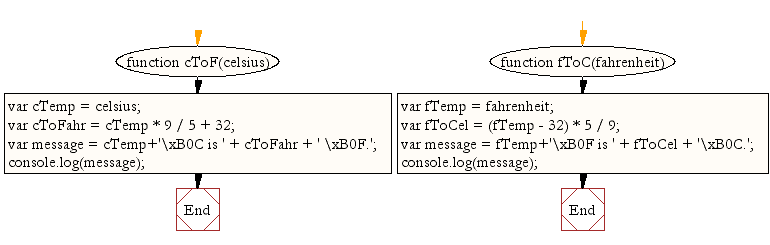# JavaScript: Convert temperatures to and from celsius, fahrenheit

## JavaScript Basic: Exercise-11 with Solution

Write a JavaScript program to convert temperatures to and from celsius, fahrenheit.

JavaScript: Fahrenheit and Centigrade Temperatures:

Fahrenheit and centigrade are two temperature scales in use today. The Fahrenheit scale was developed by the German physicist Daniel Gabriel Fahrenheit . In the Fahrenheit scale, water freezes at 32 degrees and boils at 212 degrees.

The centigrade scale, which is also called the Celsius scale, was developed by Swedish astronomer Andres Celsius. In the centigrade scale, water freezes at 0 degrees and boils at 100 degrees. The centigrade to Fahrenheit conversion formula is:

```C = (5/9) * (F - 32)
```

where F is the Fahrenheit temperature. You can also use this Web page to convert Fahrenheit temperatures to centigrade. Just enter a Fahrenheit temperature in the text box below, then click on the Convert button.Sample Solution:

HTML Code:

``````<!DOCTYPE html>
<html>
<meta charset="utf-8">
<title>Write a JavaScript program to convert temperatures to and from celsius, fahrenheit</title>
<body>
</body>
</html>
```
```

JavaScript Code:

``````function cToF(celsius)
{
var cTemp = celsius;
var cToFahr = cTemp * 9 / 5 + 32;
var message = cTemp+'\xB0C is ' + cToFahr + ' \xB0F.';
console.log(message);
}

function fToC(fahrenheit)
{
var fTemp = fahrenheit;
var fToCel = (fTemp - 32) * 5 / 9;
var message = fTemp+'\xB0F is ' + fToCel + '\xB0C.';
console.log(message);
}
cToF(60);
fToC(45);
```
```

Sample Output:

```60°C is 140 °F.
45°F is 7.222222222222222°C.
```

Explanation:

For an exact conversion (Fahrenheit to Celsius / Celsius to Fahrenheit) the following formulas can be applied :

Fahrenheit to Celsius : (°F − 32) ÷ 1.8 = °C
Celsius to Fahrenheit : (°C × 1.8) + 32 = °F

An approximate conversion between degrees Celsius and degrees Fahrenheit is as follows :

Fahrenheit to Celsius : Subtract 30 and halve the resulting number.
Celsius to Fahrenheit : Double the number and add 30.

Flowchart:ES6 Version:

``````function cToF(celsius)
{
const cTemp = celsius;
const cToFahr = cTemp * 9 / 5 + 32;
const message = `\${cTemp}\xB0C is \${cToFahr} \xB0F.`;
console.log(message);
}

function fToC(fahrenheit)
{
const fTemp = fahrenheit;
const fToCel = (fTemp - 32) * 5 / 9;
const message = `\${fTemp}\xB0F is \${fToCel}\xB0C.`;
console.log(message);
}
cToF(60);
fToC(45);
``````

Live Demo:

See the Pen JavaScript: Convert temperatures - basic-ex-11 by w3resource (@w3resource) on CodePen.

Improve this sample solution and post your code through Disqus

What is the difficulty level of this exercise?

Test your Programming skills with w3resource's quiz.

﻿

## JavaScript: Tips of the Day

Memoized function

```const add = () => {
const cache = {};
return num => {
if (num in cache) {
return 'From cache! \${cache[num]}';
} else {
const result = num + 10;
cache[num] = result;
return 'Calculated! \${result}';
}
};
};